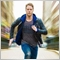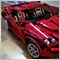# Why doenst ArrayFill work in my case?993

```input int Layers = 4              // amount of Layers the NN should consist of
input int Neuronen_L1 = 10;       // amount of neurons the first layer should consist of

double NeuronenArray[];           // here the amount of Neurons for a layer should be stored e.g. NeuronenArray = Neuronen_L1 = 10 (10 neurons in the first layer) ...     NeuronenArray = Neuronen_L2 = 10 (X neurons in the first layer)
ArrayResize(NeuronenArray,Layers);

double weight_L1[],weight_L2[],weight_L3[],weight_L4[],weight_L5[];

for (int i=0;i<Layers;i++)
{                                                   //--- place weights into the array
if (i+1 == 1){NeuronenArray[i] = Neuronen_L1;   ArrayResize(weight_L1,NeuronenArray);     Print(weight_L1);Print(weight_L1);Print(weight_L1);Print(weight_L1);Print(weight_L1);}   // print-> so that you can see
}
for (int i=0;i<Layers;i++)
{
if (i+1 == 1)
{ArrayFill(weight_L1,0,NeuronenArray[i]-1,0.5);       Print(weight_L1);Print(weight_L1);Print(weight_L1);Print(weight_L1);Print(weight_L1);} // print-> so that you can see
}```

Hey, I want to create a neural Network structure by the code above. The problem: If i try fill the weights of a layer, by FillArray() it wont change anything (for simplification i have only shown the code for the first layer).

Have you any idea why and what could be done?

the output is alway the follwoing : (the first two layers with a strange number and the rest zeros, but actually nothing has changed since the first lopp prints the same)

```2019.06.15 15:15:03.677 2014.01.01 00:00:00   9.881312916824931e-324
2019.06.15 15:15:03.677 2014.01.01 00:00:00   9.881312916824931e-324
2019.06.15 15:15:03.677 2014.01.01 00:00:00   0.0
2019.06.15 15:15:03.677 2014.01.01 00:00:00   0.0
2019.06.15 15:15:03.677 2014.01.01 00:00:00   0.0
```1765

Bayne:

Hey, I want to create a neural Network structure by the code above. The problem: If i try fill the weights of a layer, by FillArray() it wont change anything (for simplification i have only shown the code for the first layer).

Have you any idea why and what could be done?

the output is alway the follwoing : (the first two layers with a strange number and the rest zeros, but actually nothing has changed since the first lopp prints the same)

Even though your for loop goes from i=0 to Layers-1, your if condition (highlighted in green) limits execution to i==0 alone. So, end up only NeuronenArray is filled with 10, weight_L1 is resized to 10, but its values not initialized (hence giving you "strange values" which can be anything, including 0).

```for (int i=0;i<Layers;i++)
{                                                   //--- place weights into the array
if (i+1 == 1){NeuronenArray[i] = Neuronen_L1;   ArrayResize(weight_L1,NeuronenArray);     Print(weight_L1);Print(weight_L1);Print(weight_L1);Print(weight_L1);Print(weight_L1);}   // print-> so that you can see
}
```